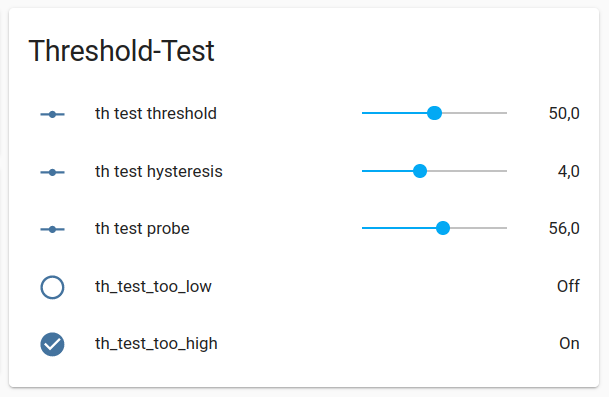# Threshold: Problem with own Implementation

As the Stock-Integration “threshold” does not allow to fetch the Values `upper`, `lower` and `hysteresis` from an `input_number`, I try to work on such a Solution, but run in to the Problem, that the Hysteresis does not work as expected.

What you will need:
The `input_numbers` to communicate with the Theshold:

``````input_number:
th_test_probe:
min: 0
max: 100
th_test_threshold:
min: 0
max: 100
th_test_hysteresis:
min: 0
max: 10
``````

A `binary_sensor`, which will reflect the current State. Choose one, depending if the Sensor should reflect “Probe is lower than Threshold” or “Probe is higher than Threshold”:

``````binary_sensor:
- platform: template
sensors:
th_test_too_low:
unique_id: th_test_too_low
value_template: >
{% set current_state = states('binary_sensor.th_test_too_low') %}
{% set probe = states('input_number.th_test_probe') | float %}
{% set th = states('input_number.th_test_threshold') | float %}
{% set hyst = states('input_number.th_test_hysteresis') | float %}
{% set lower = th - hyst %}
{% set upper = th + hyst %}
{{ probe < upper if current_state else probe < lower }}
th_test_too_high:
unique_id: th_test_too_high
value_template: >
{% set current_state = states('binary_sensor.th_test_too_high') %}
{% set probe = states('input_number.th_test_probe') | float %}
{% set th = states('input_number.th_test_threshold') | float %}
{% set hyst = states('input_number.th_test_hysteresis') | float %}
{% set lower = th - hyst %}
{% set upper = th + hyst %}
{{ probe > lower if current_state else probe > upper }}
``````

In Lovelace you may create a Card to test the whole Thing:

``````type: entities
title: Threshold-Test
entities:
- entity: input_number.th_test_threshold
- entity: input_number.th_test_hysteresis
- entity: input_number.th_test_probe
- entity: binary_sensor.th_test_too_low
- entity: binary_sensor.th_test_too_high
``````1 Like